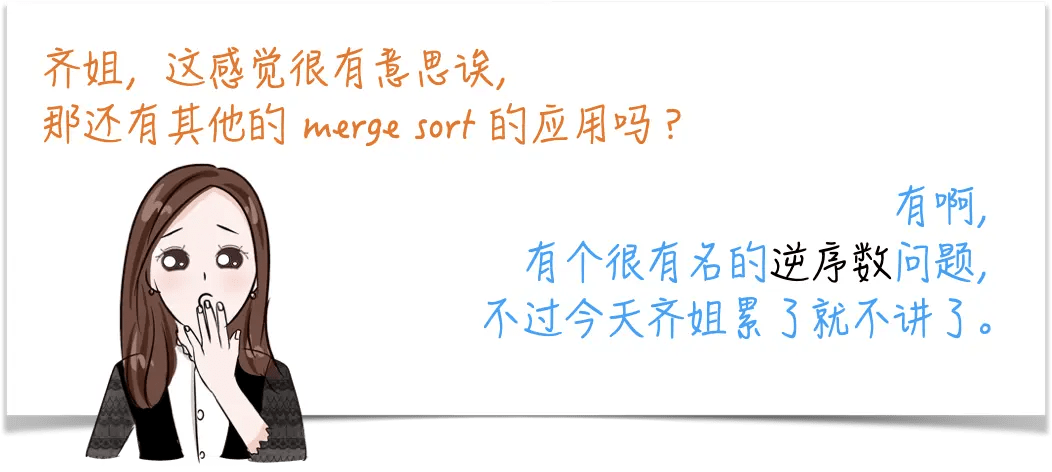# Qi Qi Comic: Sorting Algorithm (2) "Merge Sort" and "Outer Sort"

Posted May 28, 20207 min read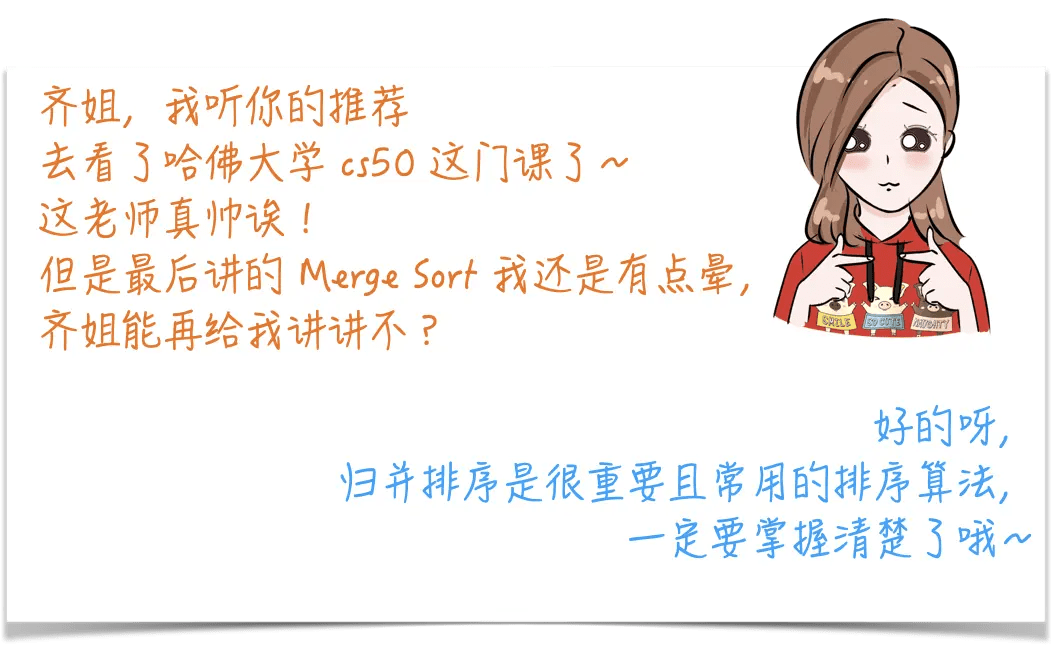Then we use the example in cs50, such as ordering a stack of rolls, how does the idea of sorting by recursion do?

1. First divide a stack of rolls into two stacks;
2. Arrange each stack in order;
3. Combine the two stacks in order.

Feeling nothing?
That's because a lot of details are omitted in the above process, let's look at them one by one.

1. The process of first dividing into two stacks, evenly divided, even and odd numbers do not matter, that is, one more and one less;
2. How are the stacks sorted?

The answer is to use the same method to arrange the order.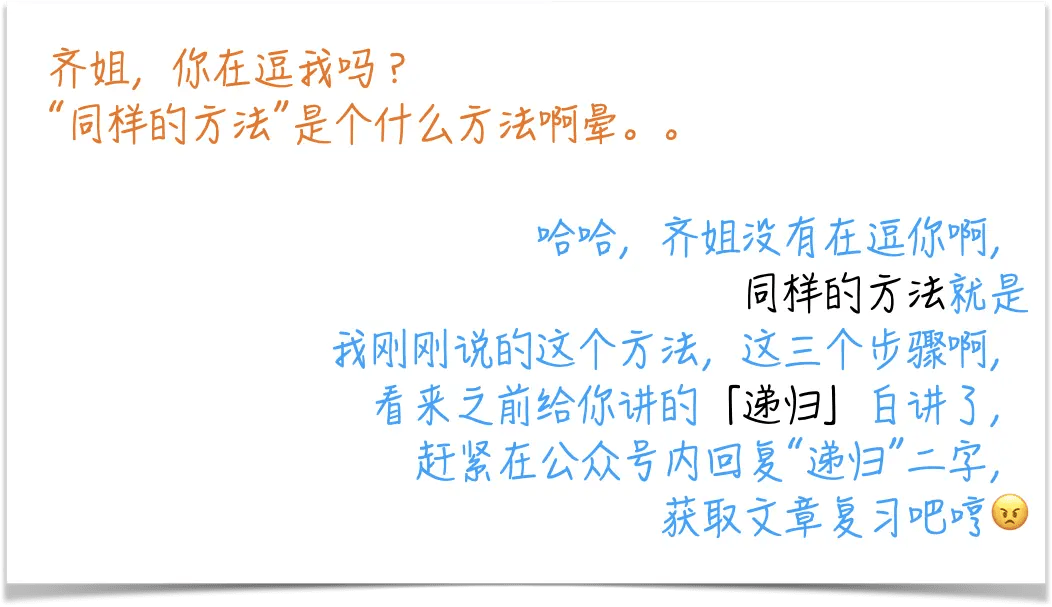1. How are the two stacked stacks combined?

You need to use two pointers and additional space here, and then draw a rainbow on the left ? draw a dragon on the right ?, no, it is a number on the left and a number on the right. After comparing the two sizes, they are placed back in the array(As for whether to put back the original array or the new array, I will talk about it later).

This is actually the idea of divide-and-conquer.
Merge sort is a very typical example.

### Divide and conquer

As the name implies:divide and conquer.

It is to decompose a big problem into similar small problems, solve these small problems, and then use the solutions of the small problems to construct the solutions of the big problems.

Does it sound similar to the previous recursion?

That s right, divide and conquer is basically recursive.

Before, we did not make a distinction. Of course now I do n t think it needs to be distinguished, but if you have to ask what is the difference between them, my understanding is:

• Recursion is a programming skill, a function that calls itself is recursive;

• The divide-and-conquer method is a problem-solving idea:

• The process of decomposing a big problem into small problems is called "point",
• The process of solving small problems is called "governance",
• The solution to small problems is often recursion.

So the three steps of the divide and conquer law are:

"Fen":Big problems are broken down into small problems;

"Governance":use the same method to solve small problems;

"Combination":Construct solutions to large problems with solutions to small problems.

Then back to our merger sorting:

"Fen":split an array into two;

"Governance":use merge sort to sort these two small arrays;

"Join":merge two small arrays into a large array.

There is still a problem here, when is it possible to solve small problems?

Answer:When there is only one element left, just return directly.
This is the recursive base case, and the answer is to be given directly.

### Old example:{5, 2, 1, 0}

Suggesting Qi's love for you ~

#### Step1.

First split it in half,
Split into two arrays:{5, 2} and {1, 0}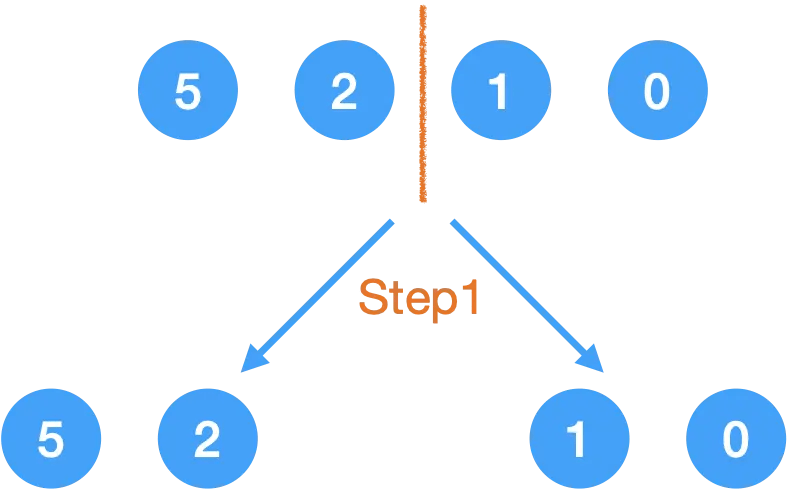#### Step2.

Did not reach the base case, so continue to decompose the big problem into small problems:Of course, although I call it Step2 on the left and right, they do not happen at the same time. I said in the recursive article that the reason is essentially caused by the Von Neumann system A CPU can only handle one thing at a time, but the reason why I write Step2 is because they occur on the same layer of the call stack. This is not demonstrated in the IDE. Students who do not understand still go to see Recur the demo in that article.

#### Step3.

This layer is an element, it is a base case, and can be returned and merged.#### Step4.

The process of merging is to arrange them in order of size. Here we use two pointers to compare, and an extra array to assist.For example, in the last step, the array has become:
{2, 5, 0, 1},
Then through the two pointers i and j, compare the size of the element pointed to by the pointer, and put the smaller one into a new array? , Then the pointer moves to the right accordingly.In fact, we have two options here:

• One is to merge from the new array to the original array,
• The other is to merge from the original array to the new array.

This depends on the type of return value required by the question; and in actual work, we often want to change the current array and sort the current array instead of returning a new array, so we take ** Instead of storing the results in a new array, merge the new array into the original array.

How to combine them specifically, you can watch the 15-second animation:

The left and right sides of the baffle are arranged separately, then the process of merging is to use two pointers, whoever has a small number, put this number in the result, and then move the pointer until one side reaches the end(out of bounds).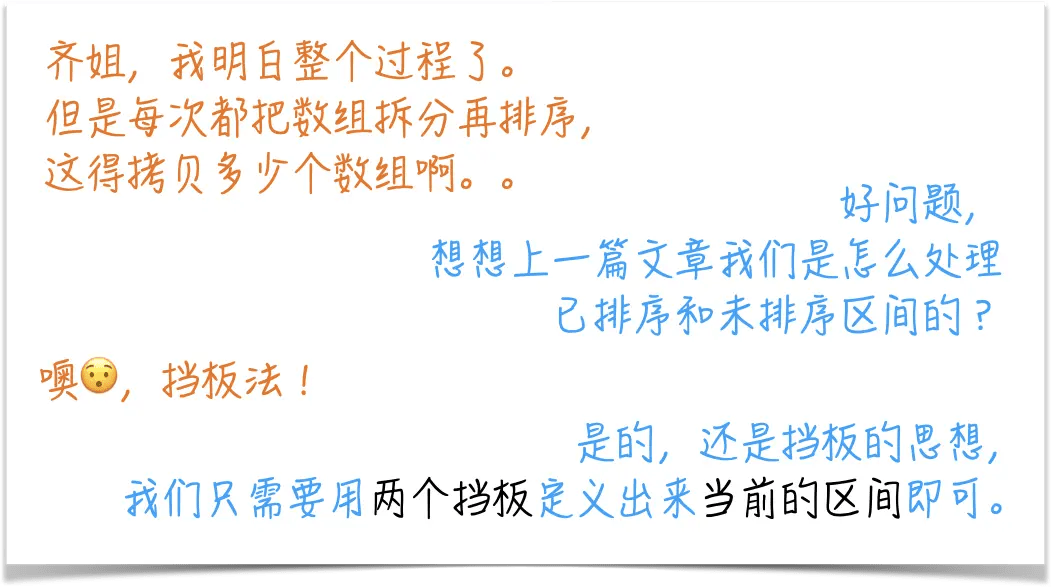``````public class MergeSort {
public void mergeSort(int []array) {
if(array == null || array.length <= 1) {
return;
}
int []newArray = new int [array.length];
mergeSort(array, 0, array.length-1, newArray);
}
private void mergeSort(int []array, int left, int right, int []newArray) {
//base case
if(left> = right) {
return;
}

//"Minute"
int mid = left +(right-left)/2;

//"Governance"
mergeSort(array, left, mid, newArray);
mergeSort(array, mid + 1, right, newArray);

//auxiliary array
for(int i = left; i <= right; i ++) {
newArray [i]= array [i];
}

//"he"
int i = left;
int j = mid + 1;
int k = left;
while(i <= mid && j <= right) {
if(newArray [i]<= newArray [j]) {//The equal sign will affect the stability of the algorithm
array [k ++]= newArray [i ++];
} else {
array [k ++]= newArray [j ++];
}
}
if(i <= mid) {
array [k ++]= newArray [i ++];
}
}
}``````

Well written, let me talk about it again:

First define the base case, otherwise it will become an infinite recursive endless loop, then here is returned when there is only one element left in the unsorted interval, that is, when the left and right baffles overlap, or when there are no elements.

#### "Minute"

Then define the small problem, find the midpoint first,

• Can we write(left + right)/2 here?
• Note , it is not allowed.

Although mathematically the same,
But write it like this,
There may be integer overflow.

#### "Governance"

In this way, we have disassembled the two left and right problems, and then use "the same method" to solve these two self-problems, so that the left and right sides are in order

• Why dare you say that both sides are in order?
• Because there is Mathematical Induction propped behind ~

Here, can it be written as:

``````mergeSort(array, left, mid-1, newArray);
mergeSort(array, mid, right, newArray);``````

In other words,

• On the left is \ [left, mid-1 ],
• On the right is \ [mid, right ],

Is this right?

Because it will cause infinite recursion.

The simplest, for example, two numbers, for example, the array is {1, 2}.

Then left = 0, right = 1, mid = 0.

The array split in this way is:

• \ [0, -1 ], \ [0, 1 ]means:
• Empty set, {1, 2}

So this way of dividing does not narrow down the problem, and does not disassemble the big problem into small problems. Such a "point" is wrong, and a stack overflow will occur.

Going deeper, the root cause is because the decimal in Java is "" round to zero ".

So it must be written here:

• On the left is \ [left, mid ],
• On the right is \ [mid + 1, right ].

The next step is the merger process.

Here we have just said that to open a new array to help merge, it is best to open in the above function, and then pass the reference down. Open one and use it repeatedly to save space.

We use two pointers:i and j point to the new array, and the pointer k points to the original array to start the moving process in the animation just now.

It should be noted that the side of the equal sign will affect the stability of this sorting algorithm. If you do n t know the stability, please go to the previous article ~

That's how it is written in my code. The pointer i refers to the element on the left. Elements that are equal will be copied first, so the elements on the left are always on the left, maintaining the relative order, so they are stable.

Finally, let's analyze the complexity of time and space:

### time complexity

The process of merge sort involves recursion, so the analysis of space-time complexity is a bit more complicated. In the previous article on "Recursion", I mentioned that the time to solve a large problem is to add up the time to solve all sub-problems. Plus the time for the merger.

Let's take a closer look at the recursive tree: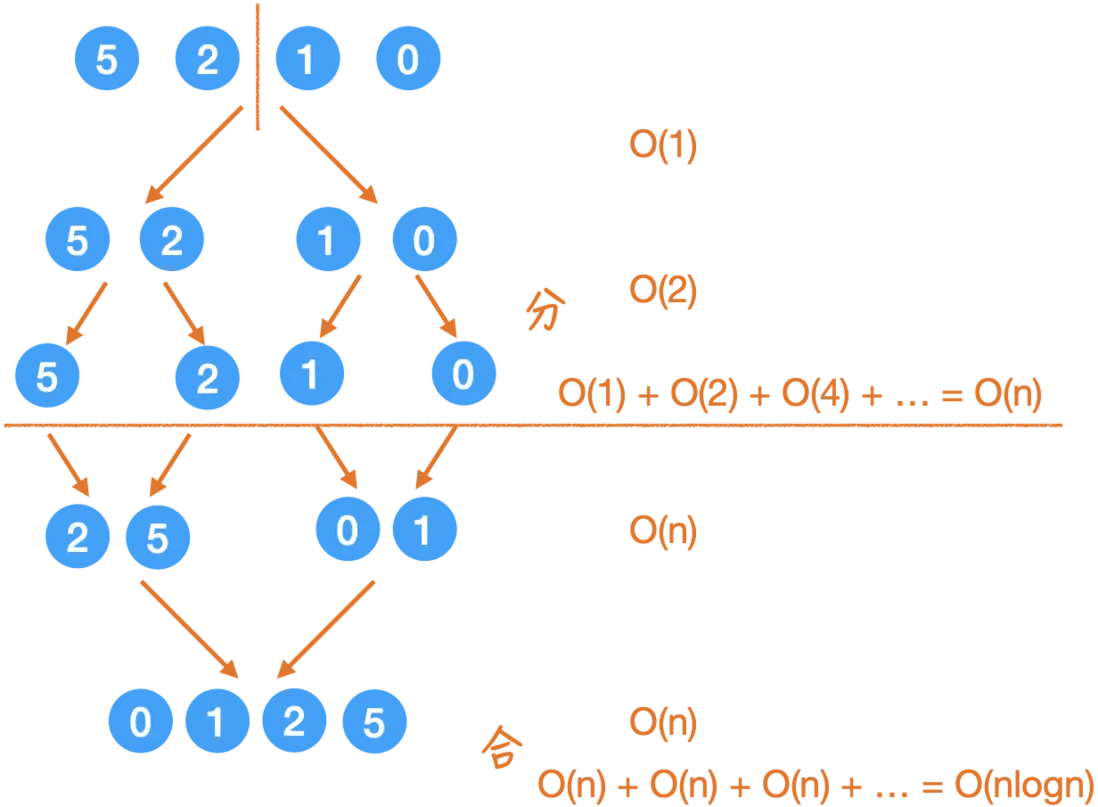Here I have written on the right:

The process of "dividing" each time depends on how many minor problems there are, it can be seen that
1, 2, 4, 8 ... increasing like this,

The process of "joining" requires two pointers to complete the process each time. The call stack of each layer adds up to O(n). There are a total of logn layers, so it is O(nlogn).

Then the total time is O(nlogn).

### Space complexity

In fact, the space complexity of the merge sort has a great relationship with how the code is written, so the space complexity I analyzed here is for my writing above.

It should be noted that the analysis of recursive space complexity cannot be directly accumulated as time complexity, because the definition of space complexity is the peak value of the use space during the running of the program, which is a peak instead The concept of accumulated value.

That is the moment when the highest used space in the call stack is actually the right-most route in the recursive tree:it must store the results of the left half of the order, and continue to arrange the right half of the total. It is O(n).

Some students said that the call stack has a logn layer, why is it not O(logn), because the space used by each layer is not O(1).

### Extended:External sort

The sorting algorithms introduced in these two sections are internal sorting algorithms, which means that the sorting process is done in memory.

However, in actual work, when the amount of data is particularly large, or larger than the memory capacity, the data cannot be put into the memory at once, and can only be placed on external storage such as a hard disk, which requires the use of External sorting algorithm algorithm to complete. A typical external sorting algorithm is External Merge Sort(External Merge Sort).

This is an interesting interview question. On the basis of the classic algorithm, plus restrictions in actual work, and the interviewer during the discussion, you can see the skill of the candidate.

To solve this problem, it is necessary to clear what are the restrictions here:

First of all, there is not enough memory. In addition, we also want to read and write hard disks as little as possible, because it is very slow.

Take the example on wiki , to sort 900MB of data, But the memory is only 100MB, so how to arrange it?

1. What is given in the wiki is to read 100MB of data into memory. I disagree, because it takes space for both sorting and fast sorting. The space complexity O(n) just said is not. The memory is full, how to run the program? Then I suggest, for example, to read 10MB of data, which is equivalent to dividing 900MB of data into 90 copies;
2. Write to disk after sorting in memory;
3. Sort the 90 pieces of data, and then 90 temporary files will be generated;
4. Use k-way merge to merge 90 files, for example, read 1MB of each file every time and get it into memory to merge, to ensure that the sum is less than the memory capacity and can ensure that the program can run.

Then this is on a machine. If the amount of data is large, such as in a distributed system, you need to use Map-Reduced for merge sorting. Interested students will continue to follow me ~